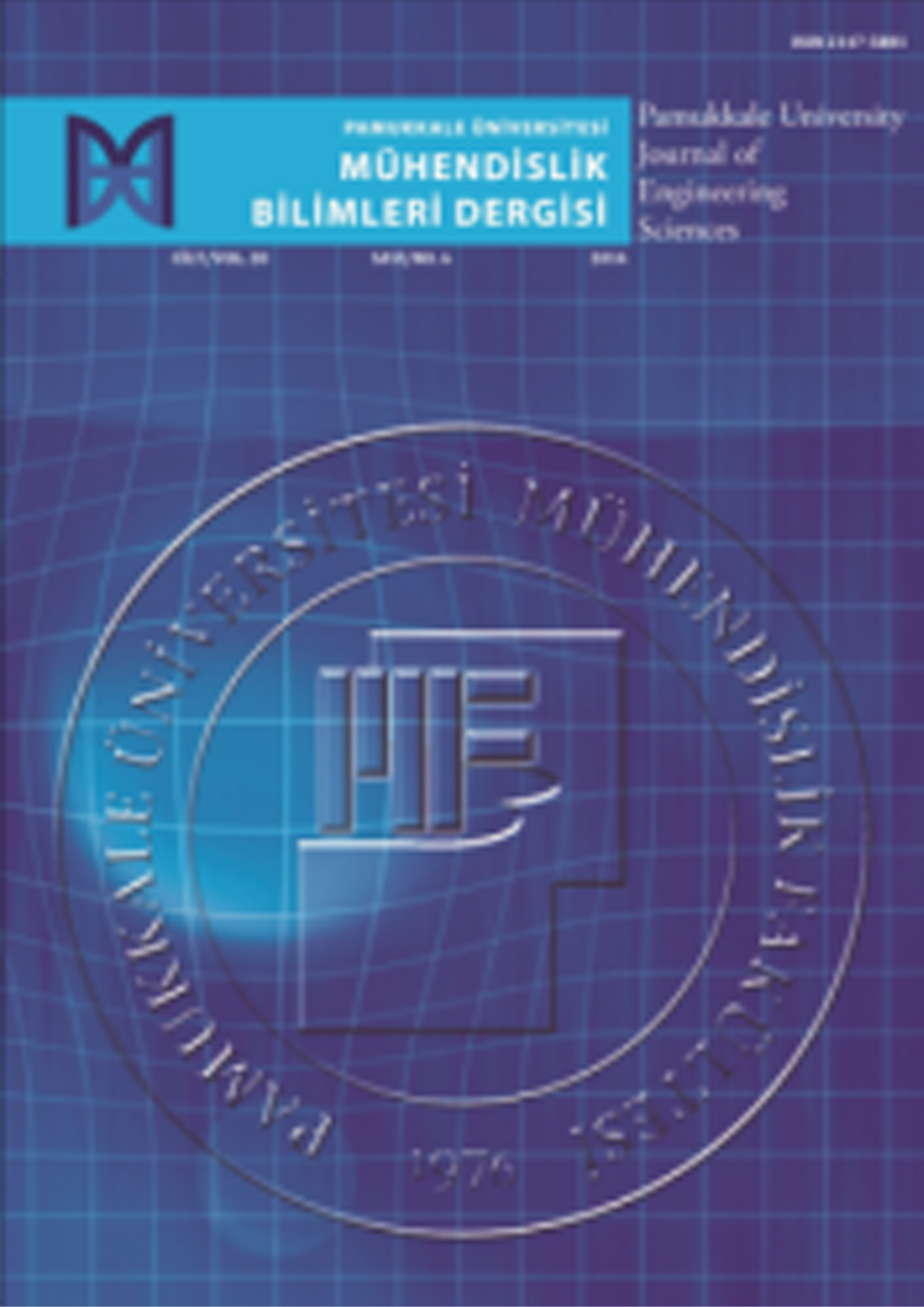E-ISSN: 2587-0351 | ISSN: 1300-2694Cilt : 28 Sayý : 3 Yýl : 2022
Pamukkale Üniversitesi Mühendislik Bilimleri Dergisi
[Pamukkale Univ Muh Bilim Derg]

## Computational assessment of external force acting on beam elastic foundation

Abdgafar Tunde Tiamiyu1, Falade Iyanda Kazeem2, Abdullahi Shuaibu Abubakar2
1Department of Mathematics, Federal University of Technology, Minna, Nigeria
2Department of Mathematics, Kano University of Science and Technology, Wudil Kano State, Nigeria

In this paper, we study some numerical methods to solve problems arising in applied sciences and engineering, primarily in beam theory. The problems of a beam on elastic foundation are usually describe with ill-posed fourth-order ordinary differential equations (ODEs). We study and formulate algorithms to aid the computation of the Exponentially Fitted Collocation Algorithm (EFCA), Hybrid Block Method (HBM), Homotopy Perturbation Algorithm (HPA), and Differential Transformation Algorithm (DTA) for the numerical solution of the ill-posed fourth-order ODEs. The formulated algorithms are further used for numerical comparison of the results. The results show that the algorithms are efficient, and numerical methods prove to be highly effective for solving beam problems.

Keywords: Ill-posed Fourth-order Beam Problems, Exponentially Fitted Collocation Algorithm (EFCA), Hybrid Block Method (HBM), Homotopy Perturbation Algorithm (HPA), Differential Transformation Algorithm (DTA)

Abdgafar Tunde Tiamiyu, Falade Iyanda Kazeem, Abdullahi Shuaibu Abubakar. Computational assessment of external force acting on beam elastic foundation. Pamukkale Univ Muh Bilim Derg. 2022; 28(3): 401-407

Sorumlu Yazar: Abdgafar Tunde Tiamiyu, Nigeria
Makale Dili: Ýngilizce